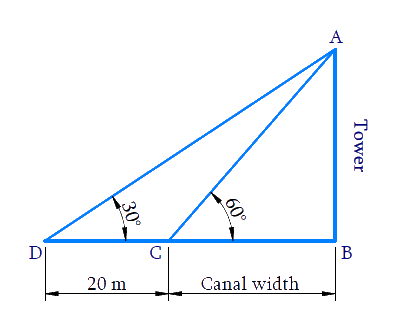# Ex.9.1 Q11 Some Applications of Trigonometry Solution - NCERT Maths Class 10

Go back to  'Ex.9.1'

## Question

A TV tower stands vertically on the bank of a canal. From a point on the other bank directly opposite the tower, the angle of elevation of the top of the tower is $$60^\circ$$. From another point $$20 \,\rm{m}$$ away from this point on the line joining this point to the foot of the tower, the angle of elevation of the top of the tower is $$30^\circ$$ see Figure. Find the height of the tower and the width of the canal.

Video Solution
Some Applications Of Trigonometry
Ex 9.1 | Question 11

## Text Solution

What is Known?

(i) The angle of elevation of the top of the tower from a point on the other bank directly opposite the tower is $$60^\circ$$

(ii) From another point $$20\,\rm{ m}$$ away from this point in (i) on the line joining this pointto the foot of the tower, the angle of elevation of the top of the tower is $$30^\circ$$

(iii)  $$CD =20\,\rm{ m}$$

What is Unknown?

Height of the tower $$=AB$$  and the width of canal $$=BC$$Reasoning:

Trigonometric ratio involving $$CD,\, BC,$$ angles and height of tower $$AB$$ is $$tan\,\theta$$.

Steps:

Considering $$\Delta ABC,$$

\begin{align} \tan 60 ^ { \circ } & = \frac { A B } { B C } \\ \sqrt { 3 } & = \frac { A B } { B C } \\ A B & = \sqrt { 3 } B C \ldots . ( 1 ) \end{align}

Considering $$\Delta ABD,$$

\begin{align}\text{tan 3}{{\text{0}}^{0}}&=\frac{AB}{BD} \\ \text{tan 3}{{\text{0}}^{0}}&=\frac{AB}{CD+BC} \\ \frac{1}{\sqrt{3}}&\!\!=\!\!\frac{BC\sqrt{3}\,}{20+BC}{From}\,(1) \\ 20+BC&=BC\sqrt{3}\times \sqrt{3} \\ 20+BC&=3BC \\ 3BC-BC&=20 \\ 2BC&=20 \\ BC&=10\end{align}

Substituting$$BC = 10 \,\rm{m}$$ in Equation ($$1$$), we get

$$\text{AB = 10}\sqrt{\text{3}}\,\rm{m}$$

Height of the tower $$\text{AB = 10}\sqrt{\text{3}}$$

Width of the canal $$BC=10 \,\rm{m}$$

Learn from the best math teachers and top your exams

• Live one on one classroom and doubt clearing
• Practice worksheets in and after class for conceptual clarity
• Personalized curriculum to keep up with school